Latest Banking jobs   »

# Quantitative Aptitude Quiz For IBPS RRB PO/Clerk Mains 2022- 16th September

Directions (1-5): Calculate Quantity I and Quantity II on the basis of the given information then compare them and answer the following questions accordingly.

Q1. Quantity I: How many days A would require to complete the work, working alone.
If two workers A and B working together completed a job in 5 days and if A worked twice as efficiently as he actually did and B worked ⅓ as efficiently as he actually did, the work would have been completed in 3 days.
Quantity II: Arun completes 2/3 of a certain job in 6 days. Lakshya can complete 1/3 of the same job in 8 days and Pulkit can complete 3/4 of the work in 12 days. All of them work together for 4 days and then Arun and Pulkit quit. How long will it take for Lakshya to complete the remaining work alone?
(a) Quantity I > Quantity II
(b) Quantity I < Quantity II
(c) Quantity I ≥ Quantity II
(d) Quantity I ≤ Quantity II
(e) Quantity I = Quantity II

Q2. Quantity I: A boat can travel ‘x’ km upstream in 4 hours and ‘y’ km downstream in 3 hours. If the speed of boat in still water is 5 km/h and the boat can travel a distance of ‘x+y’ km downstream in 4 hours, then find the speed of stream?
Quantity II: If time taken to cover (D+11) km upstream is thrice the time taken to cover (D–3) km downstream. If ratio of speed of boat in upstream to the downstream is 3 : 7 and time taken to cover (D+18) km downstream is 2.5 hours what will be the speed of current?
(a) Quantity I > Quantity II
(b) Quantity I < Quantity II
(c) Quantity I ≥ Quantity II
(d) Quantity I ≤ Quantity II
(e) Quantity I = Quantity II

Q3. Quantity I: The respective ratio between the present ages of son, mother, father and grandfather is 2 : 7 : 8 : 12. The average age of son and mother is 27 yr. What will be mother’s age after 7 yr?
Quantity II: The age of the father 5 years ago was 5 times the age of his son. At present the father’s age is 3 times that of his son. What is the present age of the father ?
(a) Quantity I > Quantity II
(b) Quantity I < Quantity II
(c) Quantity I ≥ Quantity II
(d) Quantity I ≤ Quantity II
(e) Quantity I = Quantity II

Q4. Quantity I: C’s share, if Rs. 3000 distributed among A, B and C such that A gets 2/3 rd of what B and C together get and C gets 1/2 of what A and B together get
Quantity II: Aman’s share, if Rs. 2250 is divided among three friends Aman, Bijoy and Chandra in such a way that 1/6 th of Aman’s share, 1/4 thof Bijoy’s share and 2/5 th of Chandra’s share are equal.
(a) Quantity I > Quantity II
(b) Quantity I < Quantity II
(c) Quantity I ≥ Quantity II
(d) Quantity I ≤ Quantity II
(e) Quantity I = Quantity II or No relation

Q5. A boat sails down the river for 10 km and then up the river for 6 km. The speed of the river is 1 km/hr.
Quantity I: Speed of boat in still water, if for the whole trip it takes 4 hours.
Quantity II: 4 km/hr
(a) Quantity I > Quantity II
(b) Quantity I < Quantity II
(c) Quantity I ≥ Quantity II
(d) Quantity I ≤ Quantity II
(e) Quantity I = Quantity II or No relation

Directions (6-10): The following questions are accompanied by two statements I and II. You have to determine which statements(s) is/are sufficient/necessary to answer the questions.
(a) Statement I alone is sufficient to answer the question but statement II alone is not sufficient to answer the question.
(b) Statement II alone is sufficient to answer the question but statement I alone is not sufficient to answer the question.
(c) Both the statements taken together are necessary to answer the questions, but neither of the statements alone is sufficient to answer the question.
(d) Either statement I or statement II by itself is sufficient to answer the question.
(e) Statements I and II taken together are not sufficient to answer the question.

Q6. In a building there are 40 rooms. 1/4 th of the total rooms available have both bulb and fan. And the remaining rooms have either bulb or fan but not both. Find the difference between number of rooms having bulb but not fan and the room having fan but not bulb.
I. Number of rooms having bulb is 2/3 rd of total number of rooms having fan.
II. Rooms having only fan is twice of the rooms having bulb only.

Q7. P, Q and R together can complete a work in 15 days. Find in how many days Q alone will complete that work?
I. P and Q together can complete that work in 27 days and P and R together can do that work in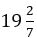days.
II. S who is 25% more efficient than that of R, takes same time as P and Q together takes to do that work.

Q8. A shopkeeper marked up an article 80% above the cost price and after providing some discount, sold it at a profit of Rs 500. Find the cost price of that article.
I. Profit is Rs 40 more than the discount provided.
II. Ratio of selling price to that of cost price is 17:12.

Q9. A, B and C entered into a partnership and the total amount invested by all the three is Rs 8100. And total profit earned by all of them is Rs. 42000. Find the profit of B if all three invested for a whole year.
I. B invested 1/3 rd of the total amount invested and ratio of profit of A to that of C is 4:5.
II. A invested 80% of the amount invested by C and B invested Rs 300 less than that of C.

Q10. In a bag there are balls of three different colors i.e. red, black and white. Find the probability of choosing a red ball from that bag?
I. Number of black ball is equal to the average of number of white and red ball in that bag and probability of choosing a white ball is 2/9.
II. Difference of the probability of choosing a black ball and the probability of choosing a red ball is 1/9.

Solutions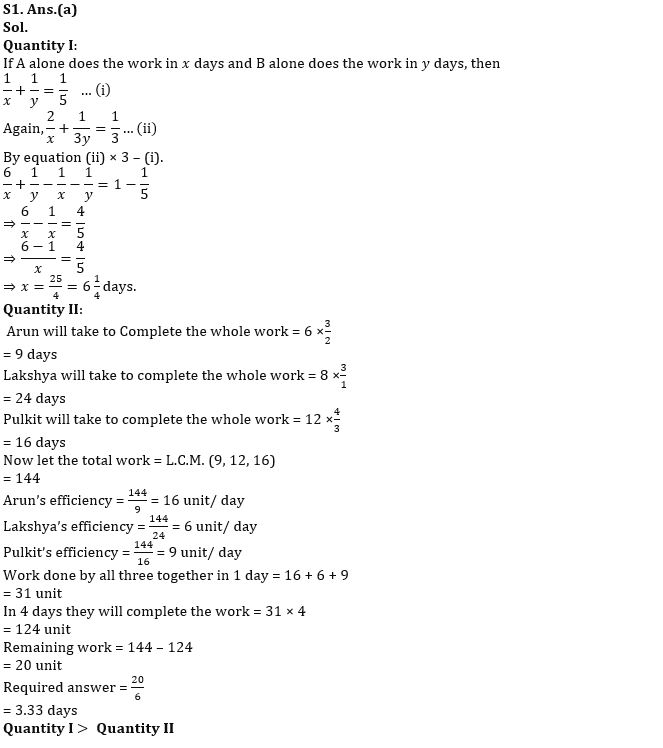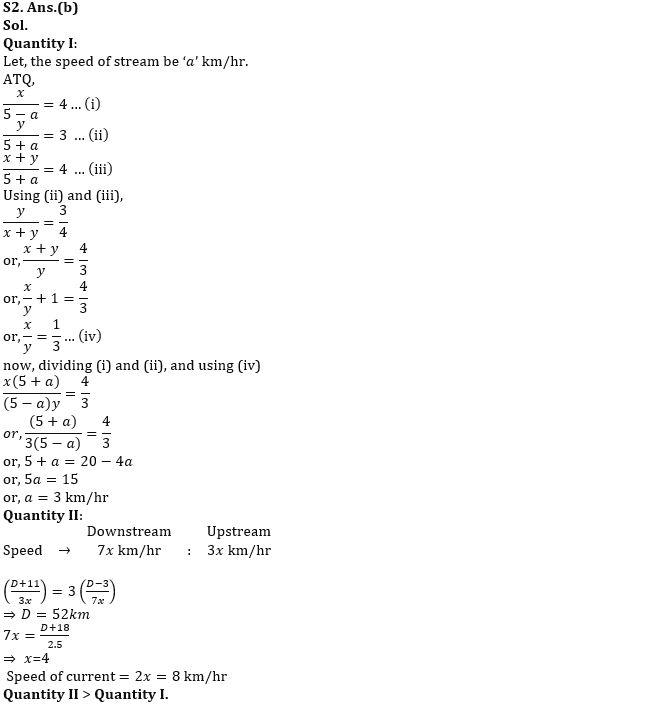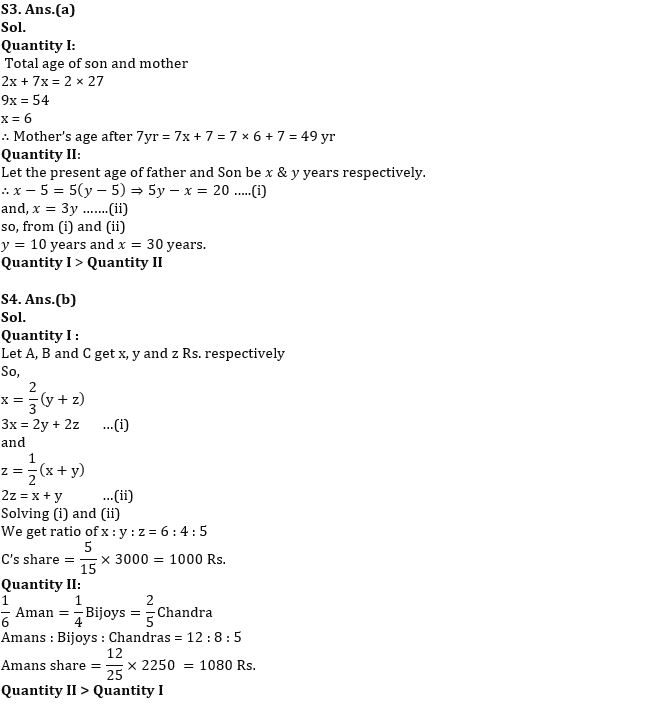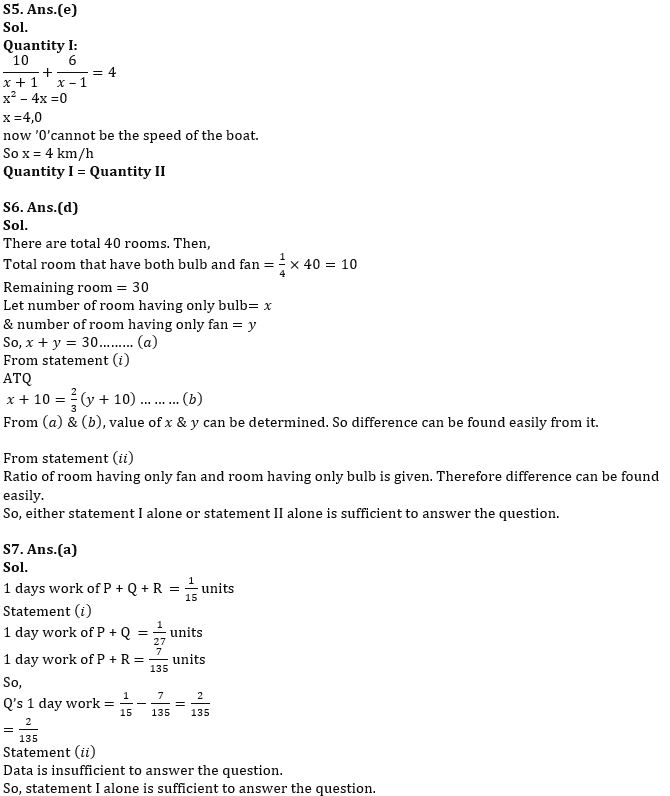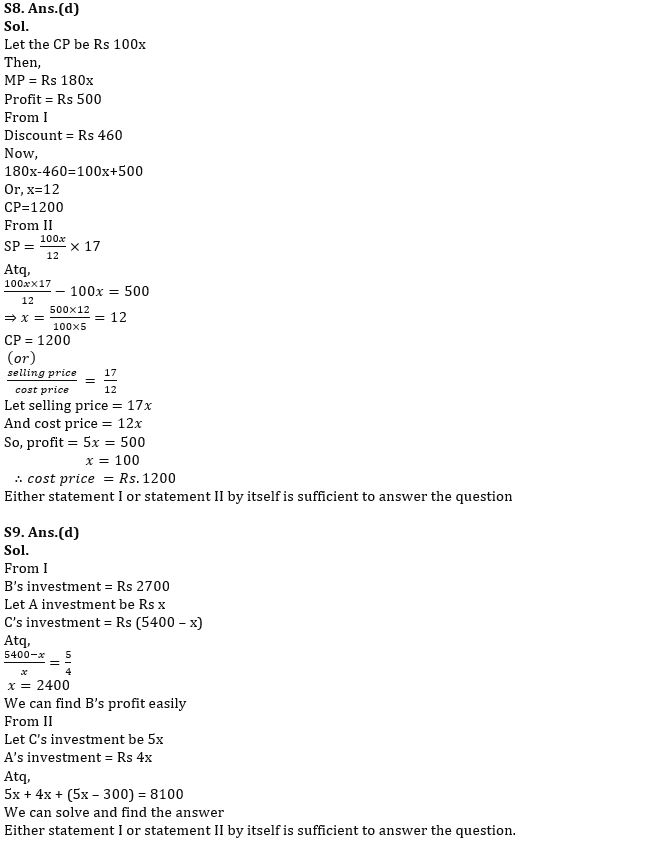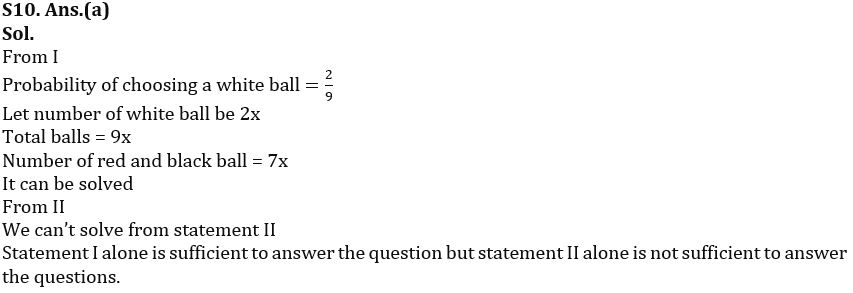#### Congratulations!Download Hindu Review of October 2021: Free PDF